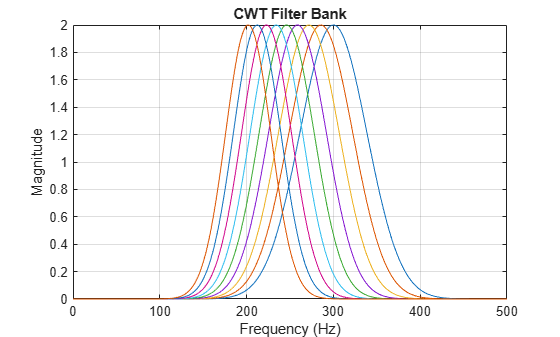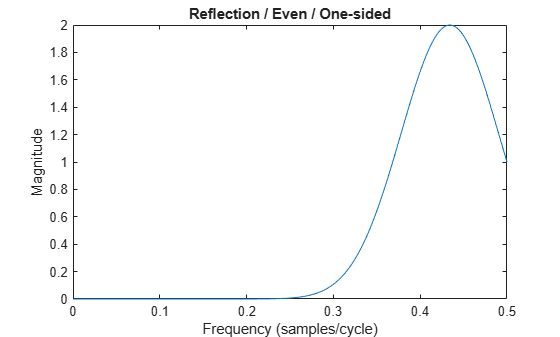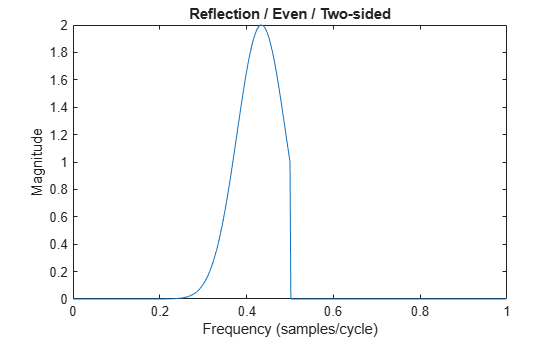# freqz

CWT filter bank frequency responses

## Syntax

``[psidft,f] = freqz(fb)``
``[___] = freqz(___,Name=Value)``
``freqz(___)``

## Description

````[psidft,f] = freqz(fb)` returns the frequency responses for the wavelet filters, `psidft`, and the frequency vector, `f`, for the continuous wavelet transform (CWT) filter bank, `fb`. Frequencies are in cycles/sample or Hz. If you specify a sampling period, the frequencies are in cycles/unit time where the time unit is the unit of the duration sampling period.The frequency responses, `psidft`, are one-sided frequency responses for the positive frequencies. For the analytic wavelets supported by `cwtfilterbank`, the frequency responses are real-valued and are equivalent to the magnitude frequency response.```

example

````[___] = freqz(___,Name=Value)` specifies one or more additional name-value arguments. For example, ```psidft = freqz(fb,FrequencyRange="twosided")``` returns the full two-sided frequency responses.```

example

````freqz(___)` with no output arguments plots the magnitude frequency responses for the CWT filter bank, `fb`.```

## Examples

collapse all

Load the Kobe earthquake data. Create a CWT filter bank with period boundary handling that you can apply to the data.

```load kobe fb = cwtfilterbank(SignalLength=numel(kobe),Boundary="periodic");```

Obtain the two-sided wavelet and scaling filter responses.

`[psidft,f] = freqz(fb,IncludeLowpass=true,FrequencyRange="twosided");`

Obtain the CWT of the data. Also obtain the scaling coefficients.

`[cfs,~,~,scalcfs] = wt(fb,kobe);`

Invert the transform using the filter bank and the scaling coefficients.

```xrec = icwt(cfs,ScalingCoefficients=scalcfs,AnalysisFilterBank=psidft); plot([kobe(:) xrec(:)]) axis tight```Obtain the maximum reconstruction error.

`norm(kobe(:)-xrec(:),'Inf')`
```ans = 2.9104e-11 ```

Create a CWT filter bank. Set the voices per octave to 14, the sampling frequency to 1000 Hz, and frequency limits to range from 200 Hz to 300 Hz.

```fb = cwtfilterbank(VoicesPerOctave=14,... SamplingFrequency=1000,FrequencyLimits=[200 300]);```

Plot the frequency responses.

`freqz(fb)`This example shows how boundary handling and signal length affect the range of frequency responses `freqz` returns.

Reflection / Even Length

Create a CWT filter bank suitable for an even-length signal. Use the default `Boundary` setting `reflection`.

```sLen = 256; fb = cwtfilterbank(SignalLength=sLen);```

Obtain the one-sided frequency responses of the filter bank. Also obtain the frequency vector.

`[psidft,f] = freqz(fb,FrequencyRange="onesided");`

Confirm the range of frequencies includes the Nyquist.

`f(end)`
```ans = 0.5000 ```

Plot the frequency response of the filter with the highest center frequency.

```plot(f,psidft(1,:)) xlabel("Frequency (samples/cycle)") ylabel("Magnitude") title("Reflection / Even / One-sided")```Obtain and plot the two-sided frequency responses. Confirm the frequency range does not include the Nyquist.

```[psidft,f] = freqz(fb,FrequencyRange="twosided"); f(end)```
```ans = 0.9980 ```
```plot(f,psidft(1,:)) xlabel("Frequency (samples/cycle)") ylabel("Magnitude") title("Reflection / Even / Two-sided")```Reflection / Odd Length

Create a CWT filter bank suitable for an odd-length signal. Use the default `Boundary` setting `reflection`.

```sLen = 255; fb = cwtfilterbank(SignalLength=sLen);```

Obtain the one-sided frequency responses of the filter bank. Confirm the range of frequencies does not include the Nyquist.

```[~,f] = freqz(fb,FrequencyRange="onesided"); f(end)```
```ans = 0.4990 ```

Periodic / Even Length

Create a CWT filter bank with periodic boundary handling suitable for an even-length signal

```sLen = 256; fb = cwtfilterbank(SignalLength=sLen,Boundary="periodic");```

Obtain the one-sided frequency responses of the filter bank. Confirm the range of frequencies does include the Nyquist.

```[~,f] = freqz(fb,FrequencyRange="onesided"); f(end)```
```ans = 0.5000 ```

Periodic / Odd Length

Create a CWT filter bank with periodic boundary handling suitable for an odd-length signal

```sLen = 255; fb = cwtfilterbank(SignalLength=sLen,Boundary="periodic");```

Obtain the one-sided frequency responses of the filter bank. Confirm the range of frequencies does not include the Nyquist.

```[~,f] = freqz(fb,FrequencyRange="onesided"); f(end)```
```ans = 0.4980 ```

## Input Arguments

collapse all

Continuous wavelet transform (CWT) filter bank, specified as a `cwtfilterbank` object.

### Name-Value Arguments

Specify optional pairs of arguments as `Name1=Value1,...,NameN=ValueN`, where `Name` is the argument name and `Value` is the corresponding value. Name-value arguments must appear after other arguments, but the order of the pairs does not matter.

Example: `psidft = freqz(fb,IncludeLowpass=true)` appends the lowpass, or scaling filter, frequency response as the final row of `psidft`.

Option to append lowpass, or scaling filter, frequency response as the final row of `psidft`, specified as one of these:

• `1` (`true`) — Include the frequency response symmetrically

• `0` (`false`) — Do not include the frequency response

For the analytic wavelets supported by `cwtfilterbank`, the scaling filter frequency response is real-valued and is equivalent to the magnitude frequency response.

Data Types: `logical`

Frequency range for the wavelet and scaling function frequency responses, specified as one of `"onesided"`, or `"twosided"`. The frequency ranges corresponding to each option are

• `"onesided"` — returns the frequency responses from [0,½] when the length of the padded filters is even and [0,½) when the length of the padded filters is odd. Padding is added when the Boundary property of the filter bank is `"reflection"`.

If a sampling frequency Fs is specified in the filter bank, the intervals become [0,Fs/2] and [0,Fs/2) respectively.

• `"twosided"` — returns the full two-sided frequency responses over the range [0,1). If a sampling frequency Fs is specified in the filter bank, the interval becomes [0,Fs).

Note

To use the wavelet and scaling filters in the inverse CWT, set Boundary in the filter bank to `"periodic"`, and use `IncludeLowpass=true` and `FrequencyRange="twosided"` in `freqz`.

## Output Arguments

collapse all

Frequency responses of a CWT filter bank, returned as a real-valued matrix. Each column of `psidft` is the response at the frequency in the corresponding element of `f`.

By default, frequency responses, `psidft`, are one-sided frequency responses for the positive frequencies. For the analytic wavelets supported by `cwtfilterbank`, the frequency responses are real-valued and are equivalent to the magnitude frequency response.

Data Types: `double`

Frequencies, in cycles/sample or hertz, returned as a real-valued vector.

If you specify a sampling period, the frequencies are in cycles/unit time, where the time unit is the unit of the duration `SamplingPeriod`.

Data Types: `double`

## Version History

Introduced in R2018a